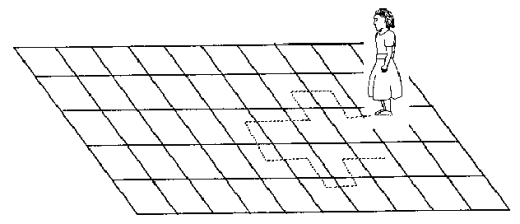# 转：时间序列预测之–ARIMA模型

## 什么是 ARIMA模型

ARIMA模型的全称叫做自回归移动平均模型，全称是(ARIMA, Autoregressive Integrated Moving Average Model)。也记作ARIMA(p,d,q)，是统计模型(statistic model)中最常见的一种用来进行时间序列 预测的模型。

### 1. ARIMA的优缺点

1.要求时序数据是稳定的（stationary），或者是通过差分化(differencing)后是稳定的。
2.本质上只能捕捉线性关系，而不能捕捉非线性关系。

### 2. 判断是时序数据是稳定的方法。

1. 稳定的数据是没有趋势(trend)，没有周期性(seasonality)的; 即它的均值，在时间轴上拥有常量的振幅，并且它的方差，在时间轴上是趋于同一个稳定的值的。
2. 可以使用Dickey-Fuller Test进行假设检验。（另起文章介绍）

### 3. ARIMA的参数与数学形式

ARIMA模型有三个参数:p,d,q。

• p–代表预测模型中采用的时序数据本身的滞后数(lags) ,也叫做AR/Auto-Regressive项
• d–代表时序数据需要进行几阶差分化，才是稳定的，也叫Integrated项。
• q–代表预测模型中采用的预测误差的滞后数(lags)，也叫做MA/Moving Average项

if d=0, yt=Ytif d=1, yt=YtYt1if d=2, yt=(YtYt1)(Yt1Yt2)=Yt2Yt1+Yt2if d=0, yt=Ytif d=1, yt=Yt−Yt−1if d=2, yt=(Yt−Yt−1)−(Yt−1−Yt−2)=Yt−2Yt−1+Yt−2

ARIMA的预测模型可以表示为：

Y的预测值 = 常量c and/or 一个或多个最近时间的Y的加权和 and/or 一个或多个最近时间的预测误差。

ARIMA用数学形式表示为：

ytˆ=μ+ϕ1yt1+...+ϕpytp+θ1et1+...+θqetqyt^=μ+ϕ1∗yt−1+…+ϕp∗yt−p+θ1∗et−1+…+θq∗et−q

,ϕARθMA其中,ϕ表示AR的系数，θ表示MA的系数

### 4.ARIMA模型的几个特例

#### 1.ARIMA(0,1,0) = random walk:Yˆt=μ+Yt1Y^t=μ+Yt−1

#### 2. ARIMA(1,0,0) = first-order autoregressive model:

p=1, d=0,q=0。说明时序数据是稳定的和自相关的。一个时刻的Y值只与上一个时刻的Y值有关。

Yˆt=μ+ϕ1Yt1.where, ϕ[1,1],Y^t=μ+ϕ1∗Yt−1.where, ϕ∈[−1,1],是一个斜率系数

#### 3. ARIMA(1,1,0) = differenced first-order autoregressive model:

p=1,d=1,q=0. 说明时序数据在一阶差分化之后是稳定的和自回归的。即一个时刻的差分（y）只与上一个时刻的差分有关。

yˆt=μ+ϕ1yt1YˆtYt1=μ+ϕ1(Yt1Yt2)Yˆt=μ+Yt1+ϕ1(Yt1Yt2)y^t=μ+ϕ1∗yt−1结合一阶差分的定义，也可以表示为：Y^t−Yt−1=μ+ϕ1∗(Yt−1−Yt−2)或者Y^t=μ+Yt−1+ϕ1∗(Yt−1−Yt−2)

#### 4. ARIMA(0,1,1) = simple exponential smoothing with growth.

p=0, d=1 ,q=1.说明数据在一阶差分后市稳定的和移动平均的。即一个时刻的估计值的差分与上一个时刻的预测误差有关。

yˆt=μ+α1et1q=1ytp=1ytyˆt=YˆtYˆt1, et1=Yt1Yˆt1,θ1=1α1Yˆt=μ+Yˆt1+α1(Yt1Yˆt1)=μ+Yt1θ1et1y^t=μ+α1∗et−1注意q=1的差分yt与p=1的差分yt的是不一样的其中，y^t=Y^t−Y^t−1, et−1=Yt−1−Y^t−1,设θ1=1−α1则也可以写成：Y^t=μ+Y^t−1+α1(Yt−1−Y^t−1)=μ+Yt−1−θ1∗et−1

#### 5. ARIMA(2,1,2)

yˆt=μ+ϕ1yt1+ϕ2yt2θ1et1θ2et2:Yˆt=μ+ϕ1(Yt1Yt2)+ϕ2(Yt2Yt3)θ1(Yt1Yˆt1)θ2(Yt2Yˆt2)y^t=μ+ϕ1∗yt−1+ϕ2∗yt−2−θ1∗et−1−θ2∗et−2也可以写成:Y^t=μ+ϕ1∗(Yt−1−Yt−2)+ϕ2∗(Yt−2−Yt−3)−θ1∗(Yt−1−Y^t−1)−θ2∗(Yt−2−Y^t−2)

#### 6. ARIMA(2,2,2)

yˆt=μ+ϕ1yt1+ϕ2yt2θ1et1θ2et2Yˆt=μ+ϕ1(Yt12Yt2+Yt3)+ϕ2(Yt22Yt3+Yt4)θ1(Yt1Yˆt1)θ2(Yt2Yˆt2)y^t=μ+ϕ1∗yt−1+ϕ2∗yt−2−θ1∗et−1−θ2∗et−2Y^t=μ+ϕ1∗(Yt−1−2Yt−2+Yt−3)+ϕ2∗(Yt−2−2Yt−3+Yt−4)−θ1∗(Yt−1−Y^t−1)−θ2∗(Yt−2−Y^t−2)

#### 7. ARIMA建模基本步骤

1. 获取被观测系统时间序列数据；
2. 对数据绘图，观测是否为平稳时间序列；对于非平稳时间序列要先进行d阶差分运算，化为平稳时间序列；
3. 经过第二步处理，已经得到平稳时间序列。要对平稳时间序列分别求得其自相关系数ACF 和偏自相关系数PACF，通过对自相关图和偏自相关图的分析，得到最佳的阶层 p 和阶数 q
4. 由以上得到的d、q、p，得到ARIMA模型。然后开始对得到的模型进行模型检验。
具体例子会在另一篇文章中给出。